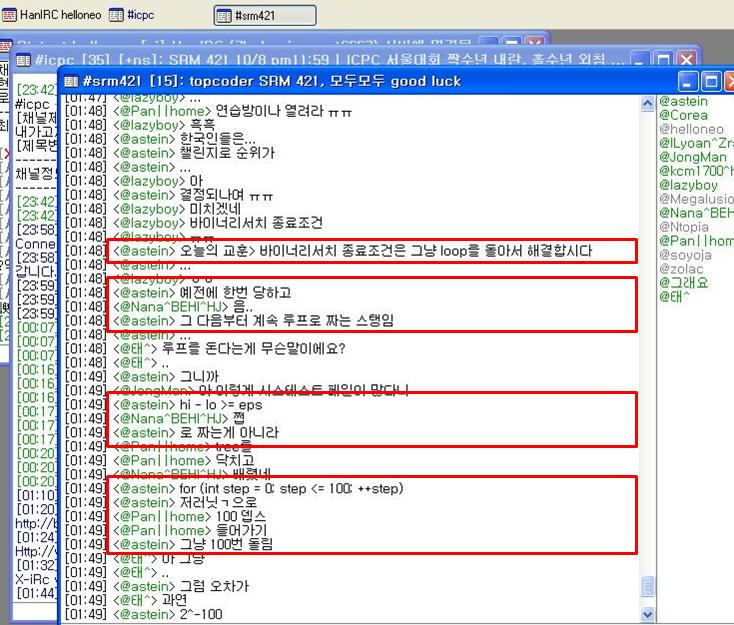## TopCoder SRM 421 Div 1

지난주 수요일 밤 12시에 열린 매치.. 오랜만에 div1에서 했는데.. 결과적으로 성적이 안좋았다.. ㅠ_ㅠ
간신히 하나 풀어서 서밋까지는 했는데 system fail.. ㅠ_ㅠ 250은 사실 좀 어이가 없었다..;
rating은 하락했지만.. 그래도 div1은 지켜냈다..;Level1 - EquilibriumPoints

이 문제는 equilibrium point를 찾는 문제이다..(????) 어떤 물체들이 일직선 상에 놓여있을때(1차원으로 가정) 오른쪽으로 발생하는 중력의 힘의 합과 왼쪽으로 발생하는 중력의 힘의 합이 같은 지점이 equilibrium point이다. 두 물체 사이에 작용하는 힘은 (질량1*질량2) / 거리^2 이다..!

갑자기 쌩뚱맞게 왠 equilibrium ??? 뭔 영화제목도 아니고.. -_-;

나는 이 문제를 다 읽자마자 바로 binary search (bisection method) 접근방법이 생각났다.. 그런데 전에도 한번 floating point number에 대해 binary search 시도했다가 time limit exceeded 난 적이 있어서.. 쉽게 시도를 못하고 삽질하다가.. 아무리 생각해도 모르겠고.. 그래서 포기하고 500점 보다가.. 500점은 더 어려운거같고.. ㅠ_ㅠ 결국 이판사판으로 250점 다시 열고 binary search를 시도했다..

챌 타임때 보니.. 다른사람도 다 나랑 같은방법으로 짠걸 보고.. 맞았구나.. 생각했는데.. 젠장.. system fail..!! 아니나다를까.. precision error로 틀린것이었다.. 이런 젠장!!! 오차범위를 10^-12로 했는데도 틀리다니!! 탑코더 정말 까다롭구만..! 다행히도 나와 같은 실수를 해서 fail한 사람이 좀 있었다..

여기서 얻을 수 있는 교훈은..?나올 수 있는 답의 범위를 우선 찾고.. 그 사이에대해서 답을 binary search 했다..

1 #include <iostream>
2 #include <cstdio>
3 #include <algorithm>
4 #include <vector>
5 #include <string>
6 #include <cmath>
7 using namespace std;
8 #define EPS 1e-12
9
10 class EquilibriumPoints {
11 public:
12
13 vector <double> getPoints(vector <int> x, vector <int> m)
14 {
15     int size;
16     int i, j, k;
17     double l, r, mid;
18     double sum1, sum2;
19     vector<double> res;
20     size = x.size();
21     for (i = 0; i < size-1; i++) {
22         l = x[i];
23         r = x[i+1];
24         k = 0;
25         while (k++ < 100) {
26             mid = (l + r) * 0.5;
27             sum1 = sum2 = 0.0;
28             for (j = 0; j <= i; j++) {
29                 sum1 += ((double)m[j]) / ((mid-x[j]) * (mid-x[j]));
30             }
31             for (j = i+1; j < size; j++) {
32                 sum2 += ((double)m[j]) / (((double)x[j]-mid) * ((double)x[j]-mid));
33             }
34             if (sum1 > sum2) {
35                 l = mid;
36             }
37             else {
38                 r = mid;
39             }
40         }
41         res.push_back(mid);
42 printf("mid = %lf\n", mid);
43     }
44     return res;
45 }
46
47 };

Level2 - CakesEqualization

input으로 케익 조각의 크기가 vector로 주어지고 maxCut이 주어진다. 케익을 최대 maxCut만큼 자를 수 있다. 한번 자른 케익 조각을 또 자를 수 있다. 이렇게 해서 가장 큰 조각과 가장 작은 조각의 차이를 최소로 하고싶다. 그 최소값 구하기

이 문제는 일종의 greedy로 해결할 수 있다..
우선 가장 큰 케익을 자르는것을 원칙으로하는데.. 그렇다고 무조건 2등분을 하는것은 아니다.
예) {100, 300}, 2 => 300을 3등분을 해야 답이 나온다..

모든 케익 C[i] 에 대해서 각각 P[i] 등분을 한다고 생각하면 된다..
그래서 C[i] / P[i] 값이 가장 큰것에 대해서 P[i] 값을 1씩 증가시켜주면 된다..
구현은 매 iteration마다 sort해도 되고.. PQ 비스무리한 자료구조를 써도 된다..~

그리고.. precesion error에도 주의해야한다.. OTL

사실 Live 3635 - Pie 문제와 비스무리한데.. 저 문제를 풀어놓고도 못풀다니.. ㅠ_ㅠ

1 #include <iostream>
2 #include <cstdio>
3 #include <algorithm>
4 #include <vector>
5 #include <string>
6 #include <set>
7 #include <cmath>
8 using namespace std;
9 #define min(x, y) ((x) > (y) ? (y) : (x))
10
11 typedef struct _d {
12     int no, den;
13 } DATA;
14
15 bool operator < (const DATA& a, const DATA& b)
16 {
17     double x, y;
18     x = (double)a.no / (double)a.den;
19     y = (double)b.no / (double)b.den;
20     return y < x;
21 }
22
23 class CakesEqualization {
24 public:
25
26 double fairDivision(vector <int> weights, int maxCuts)
27 {
28     int size, i;
29     double min1, x, y;
30     DATA d1;
31     multiset<DATA> s;
32     multiset<DATA>::iterator it;
33
34     size = weights.size();
35     for (i = 0; i < size; i++) {
36         d1.no = weights[i];
37         d1.den = 1;
38         s.insert(d1);
39     }
40     it = s.begin();
41     x = (double)it->no / (double)it->den;
42     it = s.end();
43     it--;
44     y = (double)it->no / (double)it->den;
45     min1 = x - y;
46     for (i = 0; i < maxCuts; i++) {
47         it = s.begin();
48         d1 = *it;
49         s.erase(it);
50         d1.den++;
51         s.insert(d1);
52         it = s.begin();
53         x = (double)it->no / (double)it->den;
54         it = s.end();
55         it--;
56         y = (double)it->no / (double)it->den;
57         min1 = min(min1, fabs(x - y));
58     }
59     return min1;
60 }
61
62 };

Level3 - TeamManagement

to be updated..

#### 'Problem Solving > TopCoder logs' 카테고리의 다른 글

 TopCoder SRM 432 Div 1  (0) 2009.01.07 2008.12.03 2008.11.25 2008.11.13 2008.10.19 2008.10.12 2008.10.03 2008.09.21 2008.09.13 2008.09.07 2008.08.17